Next: Axisymmetric Velocity Fields Up: Axisymmetric Incompressible Inviscid Flow Previous: Axisymmetric Flow

# Stokes Stream Function

Consider a fixed pointlying on the symmetry axis, and an arbitrary point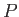. Let us jointovia two different curves,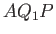and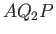, that both lie in the same plane. (See Figure 7.1.) We shall refer to this plane as the meridian plane. The position of a given point in the meridian plane can be specified either in terms of the cylindrical coordinates (,), or the spherical coordinates (,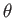). If the meridian curvesandrotate about the symmetry axis then closed surfaces are formed. Assuming that the flow pattern is incompressible, the flux of fluid from right to left (in Figure 7.1) across the surface generated bymust match that in the same direction across the surface generated by. Let us denote the flux across either of these surfaces by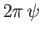. Here,is known as the Stokes stream function. If we keepfixed, and replaceby any other meridian curve joiningto, then the value ofis clearly unaltered. Thus, the stream functiondepends on the position of the arbitrary point, and, possibly, on that of the fixed point. In fact, if we take another fixed point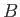on the symmetry axis, and draw the meridian curve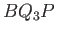, then the flux across the surface generated bywill be the same as that across the surface generated by, because, by symmetry, there is no flow across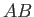. (See Figure 7.1.) It follows that the value ofdoes not depend on the particular fixed point that is used in its definition, as long as this point lies on the symmetry axis. Hence, we conclude that the value of the stream function atdepends solely on the position of. Furthermore, iflies on the axis then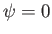.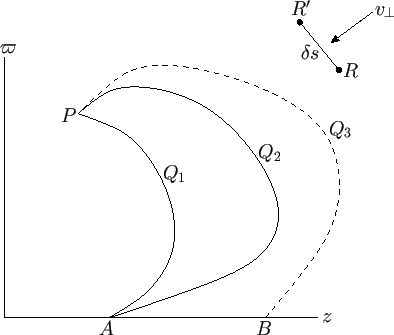Consider two neighboring points,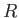and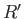, lying in the meridian plane. (See Figure 7.1.) The flux from right to left across the surface generated by revolving any line joiningtoabout the symmetry axis is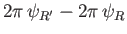. If the distance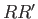takes the infinitesimal value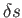then we can write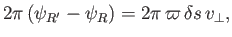(7.1)

where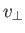is the normal flow velocity (from right to left) across the straight-linein the meridian plane. (See Figure 7.1.) It follows that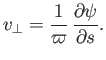(7.2)

In particular, if we suppose that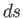is, in turn, equal to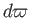,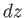,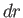, and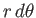then we obtain the following expressions for the in-plane velocity components in cylindrical and spherical coordinates: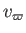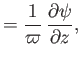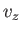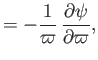(7.3)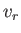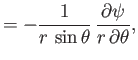(7.4)Next: Axisymmetric Velocity Fields Up: Axisymmetric Incompressible Inviscid Flow Previous: Axisymmetric Flow
Richard Fitzpatrick 2016-03-31